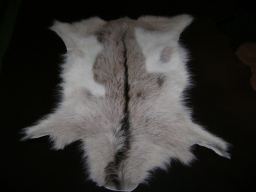# Calculate 27351

There were goats and chickens in the yard. The number of all heads was 15. The number of all legs was 40. Calculate how many hens there were. Every animal has all its legs.

a =  5
b =  10

### Step-by-step explanation:

a+b=15
4a+2b=40

a+b=15
4·a+2·b=40

a+b = 15
4a+2b = 40

Pivot: Row 1 ↔ Row 2
4a+2b = 40
a+b = 15

Row 2 - 1/4 · Row 1 → Row 2
4a+2b = 40
0.5b = 5

b = 5/0.5 = 10
a = 40-2b/4 = 40-2 · 10/4 = 5

a = 5
b = 10

Our linear equations calculator calculates it.Did you find an error or inaccuracy? Feel free to write us. Thank you!

Tips for related online calculators
Do you have a linear equation or system of equations and looking for its solution? Or do you have a quadratic equation?